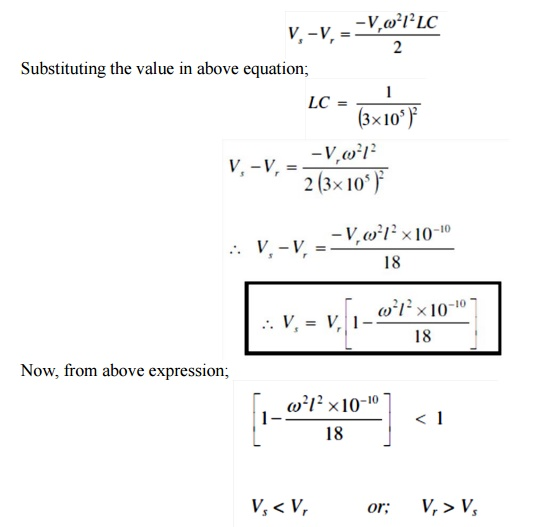Home | | Transmission and Distribution | Ferranti Effect

# Ferranti Effect

A long transmission line draws a substantial quantity of charging current. If such a line is open circuited or very lightly loaded at the receiving end, the voltage at receiving end may become greater than voltage at sending end. This is known as Ferranti Effect.

FERRANTI EFFECT

A long transmission line draws a substantial quantity of charging current. If such a line is open circuited or very lightly loaded at the receiving end, the voltage at receiving end may become greater than voltage at sending end. This is known as Ferranti Effect and is due to the voltage drop across the line inductance (due to charging current) being in phase with the sending end voltages. Therefore both capacitance and inductance is responsible to produce this phenomenon The capacitance (and charging current) is negligible in short line but significant in medium line and appreciable in long line. Therefore this phenomenon occurs in medium and long lines.

Represent line by equivalent π model.Line capacitance is assumed to be concentrated at the receiving end. OM = receiving end voltage Vr

OC = Current drawn by capacitance = Ic

MN = Resistance drop

NP = Inductive reactance drop Therefore;

OP = Sending end voltage at no load and is less than receiving end voltage (Vr)

Since, resistance is small compared to reactance; resistance can be neglected in calculating Ferranti effect.

From π model,i.e. receiving end voltage is greater than sending end voltage and this effect is called Ferranti Effect. It is valid for open circuit condition of long line.

Study Material, Lecturing Notes, Assignment, Reference, Wiki description explanation, brief detail
Transmission and Distribution : Modelling and Performance of Transmission Lines : Ferranti Effect |